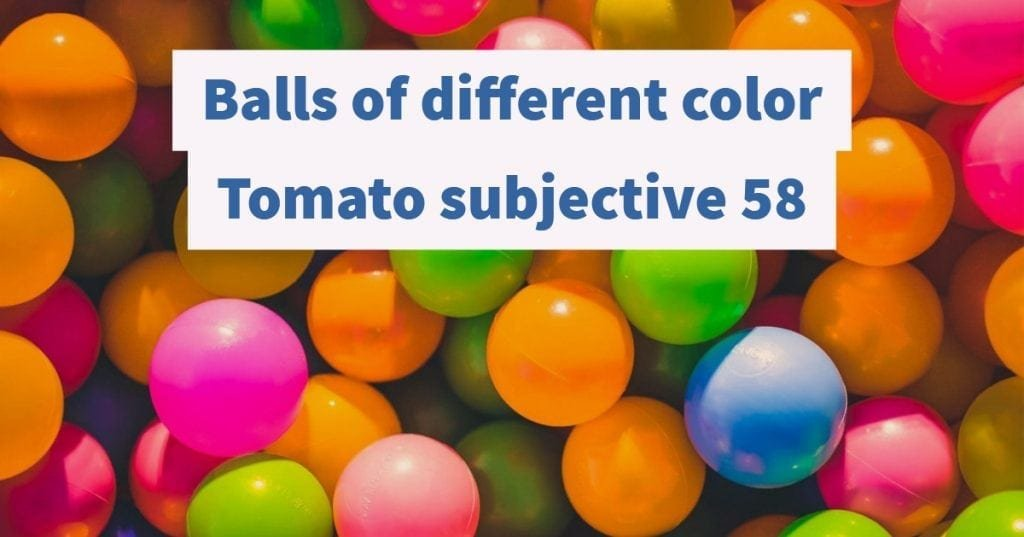How Cheenta works to ensure student success?
Explore the Back-Story

# Test of Mathematics Solution Subjective 58 - Balls of Different ColorThis is a Test of Mathematics Solution Subjective 58 (from ISI Entrance). The book, Test of Mathematics at 10+2 Level is Published by East West Press. This problem book is indispensable for the preparation of I.S.I. B.Stat and B.Math Entrance.

Also visit: I.S.I. & C.M.I. Entrance Course of Cheenta

## Problem

In a certain game, 30 balls of k, different colours are kept inside a sealed box .You are told only the value of k,but not the number of balls of each colour . Based on this, you have to guess whether it is possible to split the balls into 10 groups of each, such that in each group the three balls are of different colours.Your answer is to be a simple YES or NO.You win or loss a point according as your guess correct or not.For what values of k, you can say NO and be sure of winning?For what values of k, you can say YES and be sure of winning?Justify your solution.

## Solution:

(i) No & sure of winning :- 1,2.

Justification: If colours are there the 3 balls cannot be of different colours & if ${{k} \ge {3}}$ then there exists a possibility of splitting into two groups of 3 each in each group 3 balls are of different colours.

For k = 3 take 10 ball of each colour & the splitting is possible.

(ii) Yes & sure of winning 21,...,30

## Justification:

By we can say that number of ball of a certain colour is at most two.So we can keep them in different boxes.For ${{k} \le {20}}$ is not possible .

For example take (k-1) balls of different colours & (30-k+1) ball of different colour. Now by (30-k+1) ball of same colour are placed in two groups.So at least one group contain more than one ball of same colours.This is a Test of Mathematics Solution Subjective 58 (from ISI Entrance). The book, Test of Mathematics at 10+2 Level is Published by East West Press. This problem book is indispensable for the preparation of I.S.I. B.Stat and B.Math Entrance.

Also visit: I.S.I. & C.M.I. Entrance Course of Cheenta

## Problem

In a certain game, 30 balls of k, different colours are kept inside a sealed box .You are told only the value of k,but not the number of balls of each colour . Based on this, you have to guess whether it is possible to split the balls into 10 groups of each, such that in each group the three balls are of different colours.Your answer is to be a simple YES or NO.You win or loss a point according as your guess correct or not.For what values of k, you can say NO and be sure of winning?For what values of k, you can say YES and be sure of winning?Justify your solution.

## Solution:

(i) No & sure of winning :- 1,2.

Justification: If colours are there the 3 balls cannot be of different colours & if ${{k} \ge {3}}$ then there exists a possibility of splitting into two groups of 3 each in each group 3 balls are of different colours.

For k = 3 take 10 ball of each colour & the splitting is possible.

(ii) Yes & sure of winning 21,...,30

## Justification:

By we can say that number of ball of a certain colour is at most two.So we can keep them in different boxes.For ${{k} \le {20}}$ is not possible .

For example take (k-1) balls of different colours & (30-k+1) ball of different colour. Now by (30-k+1) ball of same colour are placed in two groups.So at least one group contain more than one ball of same colours.

### Knowledge Partner